Related Articles
ML | Determine the optimal value of K in K-Means Clustering
• Last Updated : 26 Jan, 2019

Prerequisite: K-Means Clustering | Introduction

There is a popular method known as elbow method which is used to determine the optimal value of K to perform the K-Means Clustering Algorithm. The basic idea behind this method is that it plots the various values of cost with changing k. As the value of K increases, there will be fewer elements in the cluster. So average distortion will decrease. The lesser number of elements means closer to the centroid. So, the point where this distortion declines the most is the elbow point.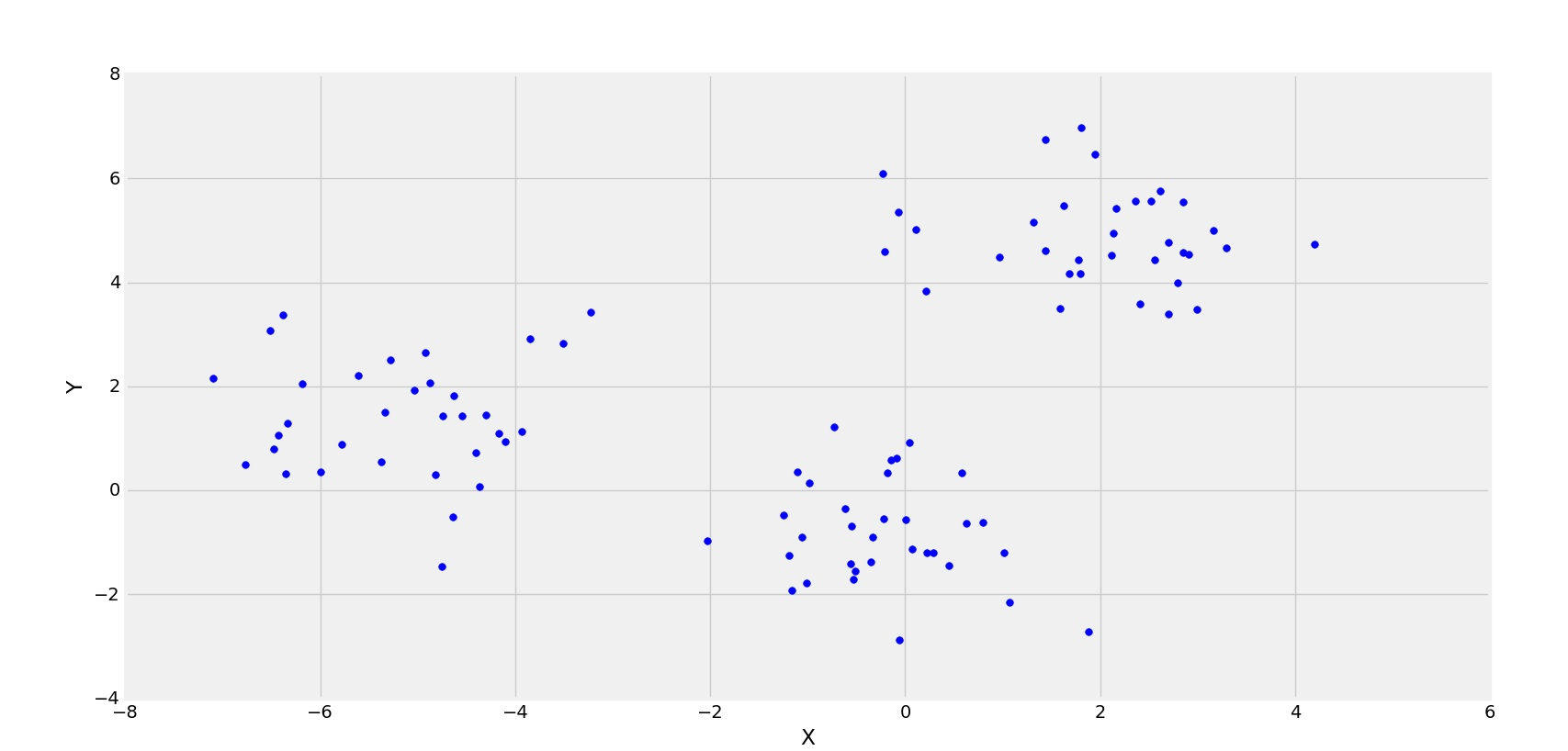3 clusters are forming

In the above figure, its clearly observed that the distribution of points are forming 3 clusters. Now, let’s see the plot for the squared error(Cost) for different values of K.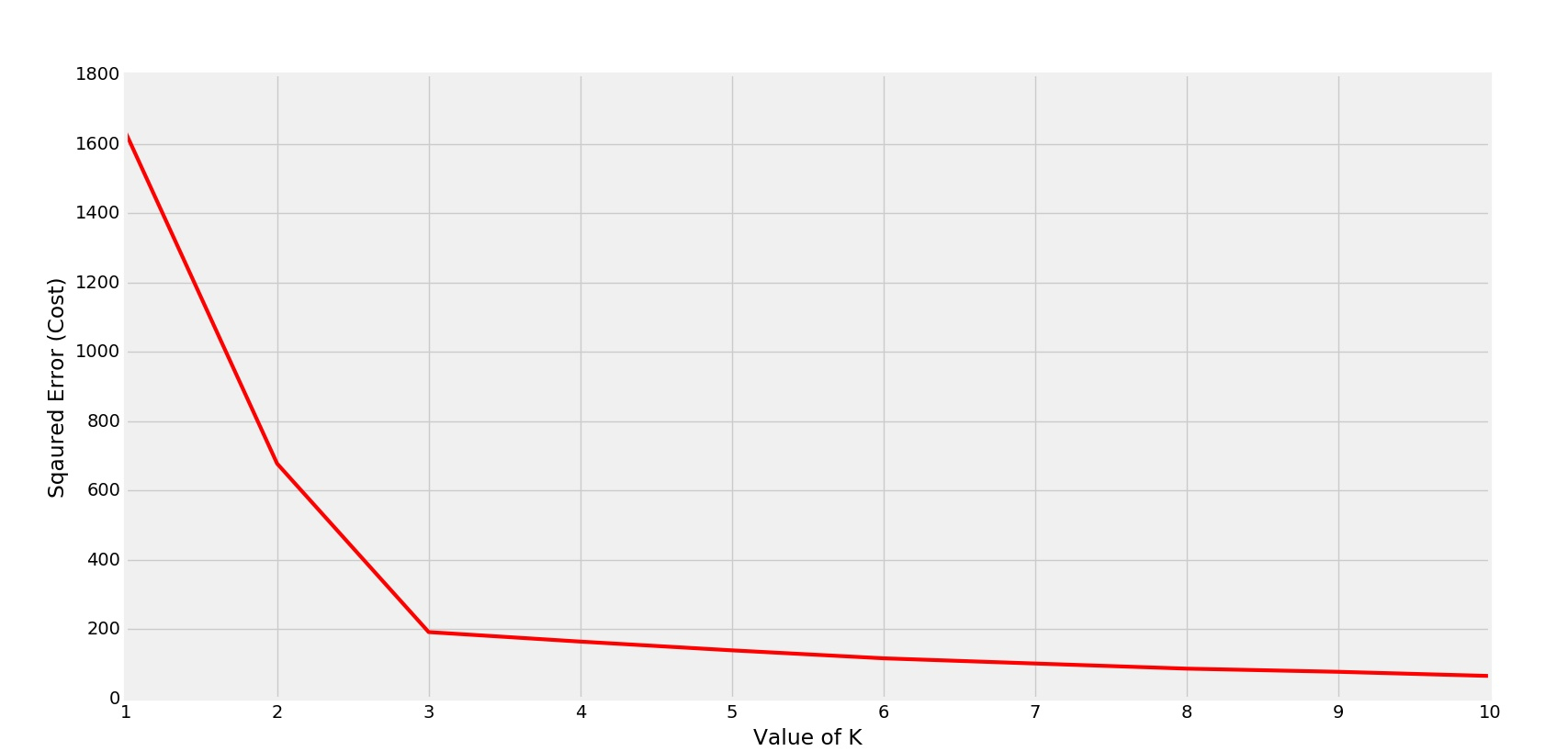Elbow is forming at K=3

Clearly the elbow is forming at K=3. So the optimal value will be 3 for performing K-Means.

Another Example with 4 clusters.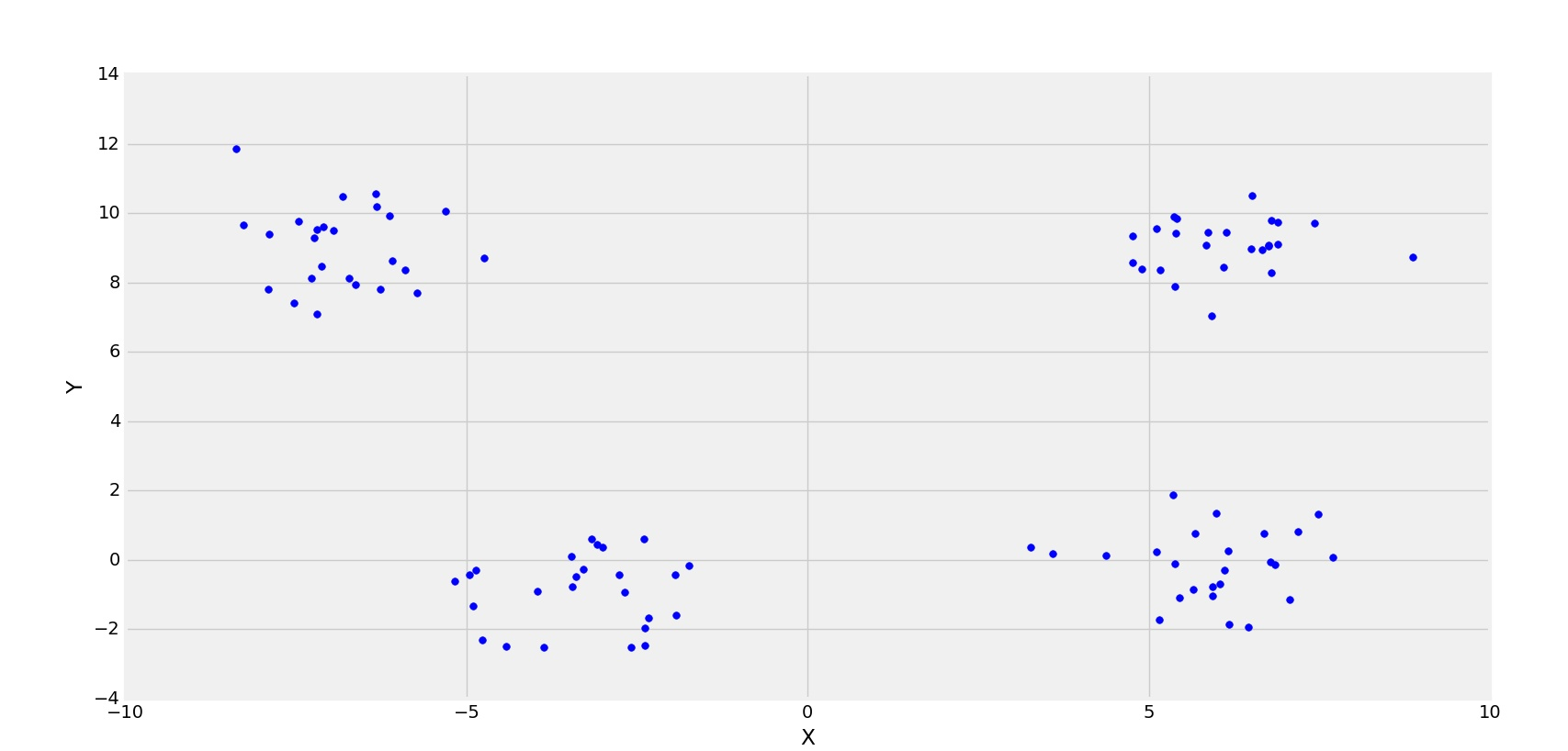4-clusters

Corresponding Cost graph-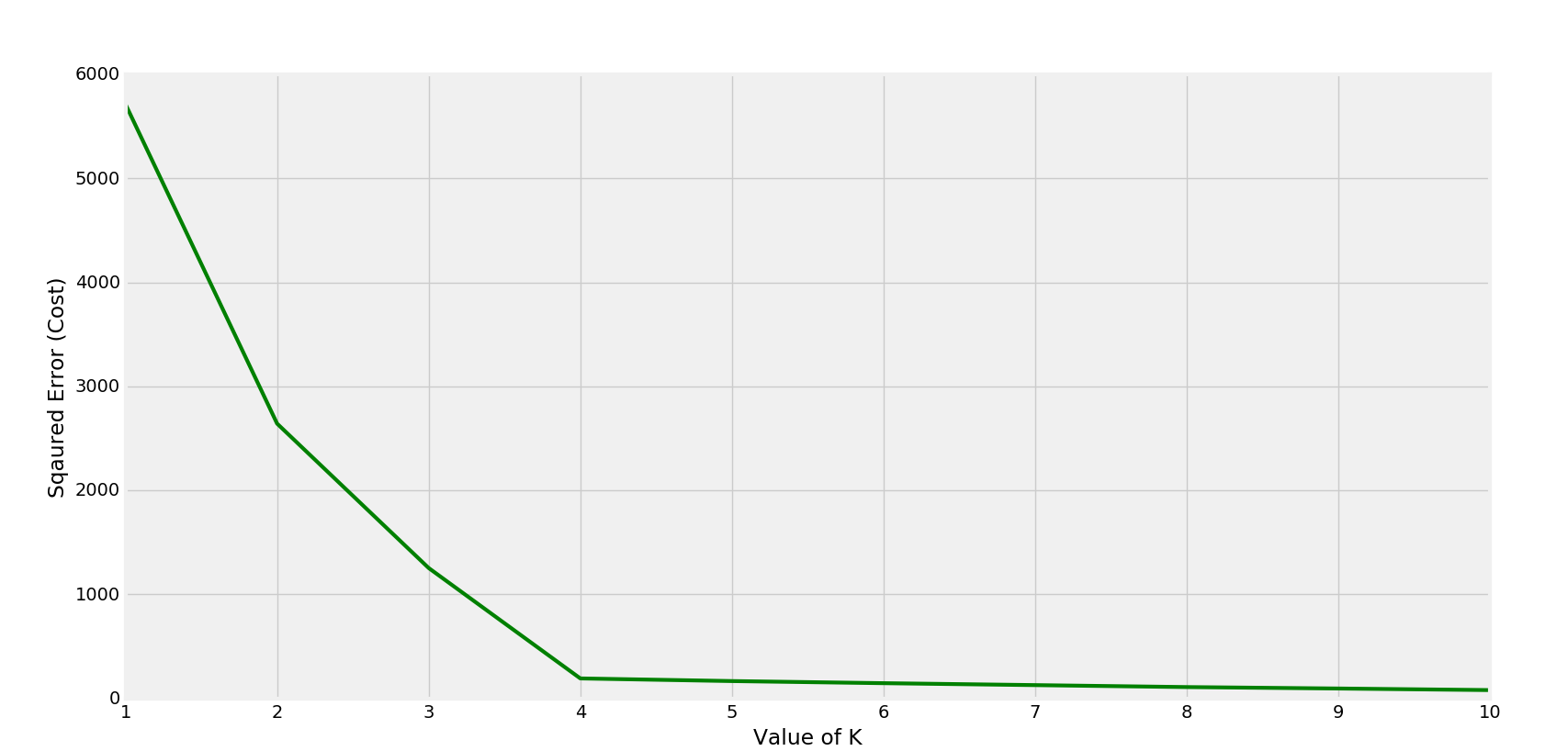Elbow is forming at K=4

In this case the optimal value for k would be 4. (Observable from the scattered points).

Below is the Python implementation:

 `import` `matplotlib.pyplot as plt ``from` `matplotlib ``import` `style``from` `sklearn.cluster ``import` `KMeans``from` `sklearn.datasets.samples_generator ``import` `make_blobs`` ` `style.use(``"fivethirtyeight"``)`` ` `# make_blobs() is used to generate sample points``# around c centers (randomly chosen)``X, y ``=` `make_blobs(n_samples ``=` `100``, centers ``=` `4``, ``                ``cluster_std ``=` `1``, n_features ``=` `2``)``                 ` `plt.scatter(X[:, ``0``], X[:, ``1``], s ``=` `30``, color ``=``'b'``)`` ` `# label the axes``plt.xlabel(``'X'``)``plt.ylabel(``'Y'``)`` ` `plt.show()``plt.clf() ``# clear the figure`

Output: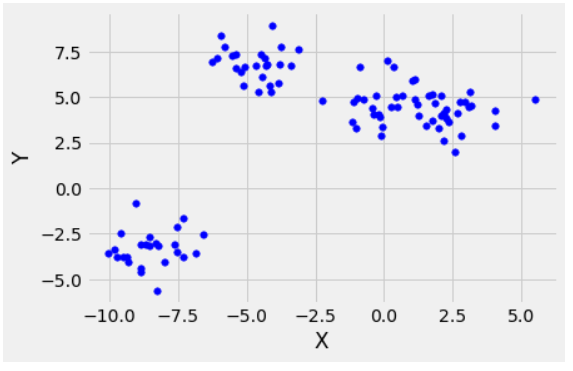`cost ``=``[]``for` `i ``in` `range``(``1``, ``11``):``    ``KM ``=` `KMeans(n_clusters ``=` `i, max_iter ``=` `500``)``    ``KM.fit(X)``     ` `    ``# calculates squared error``    ``# for the clustered points``    ``cost.append(KM.inertia_)     `` ` `# plot the cost against K values``plt.plot(``range``(``1``, ``11``), cost, color ``=``'g'``, linewidth ``=``'3'``)``plt.xlabel(``"Value of K"``)``plt.ylabel(``"Sqaured Error (Cost)"``)``plt.show() ``# clear the plot`` ` `# the point of the elbow is the ``# most optimal value for choosing k`

Output: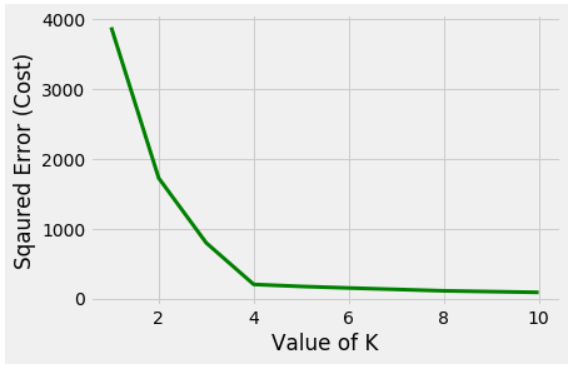My Personal Notes arrow_drop_up